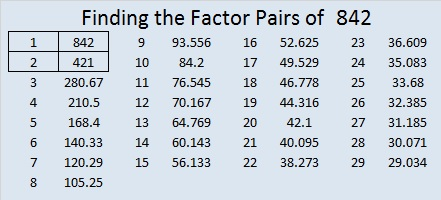# 842 and Level 6Print the puzzles or type the solution on this excel file: 10-factors-835-842

29² + 1² = 842

That means 842 is the hypotenuse of a Pythagorean triple:

• 58-840-842, calculated from 2(29)(1), 29² – 1², 29² + 1²

842 is repdigit 222 in BASE 20 because 2(20²) + 2(20¹) + 2(20º) = 842

• 842 is a composite number.
• Prime factorization: 842 = 2 × 421
• The exponents in the prime factorization are 1 and 1. Adding one to each and multiplying we get (1 + 1)(1 + 1) = 2 × 2 = 4. Therefore 842 has exactly 4 factors.
• Factors of 842: 1, 2, 421, 842
• Factor pairs: 842 = 1 × 842 or 2 × 421
• 842 has no square factors that allow its square root to be simplified. √842 ≈ 29.017236This site uses Akismet to reduce spam. Learn how your comment data is processed.